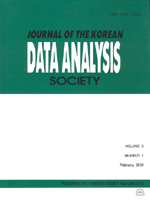상세검색
최근 검색어 전체 삭제
다국어입력
즐겨찾기0KCI등재 학술저널

# A Simulation Study on the Prediction Ability of Biased Regression Methods

• 등재여부 : KCI등재
• 2002.12
• 361 - 371 (11 pages)

This article examines three biased methods used for predictive modelling: ridge regression, principal component regression and partial least squares regression. A Monte Carlo study is performed to compare the prediction ability of these methods along with ordinary least squares. 48 different situations are considered and the root mean squared error of prediction is computed by using leave-one-out cross-validation for the comparison. Performance of the four methods are compared in detail.

1. Introduction

2. Background

3. Simulation Study

4. Results of the Simulation Study

5. Conclusions

References